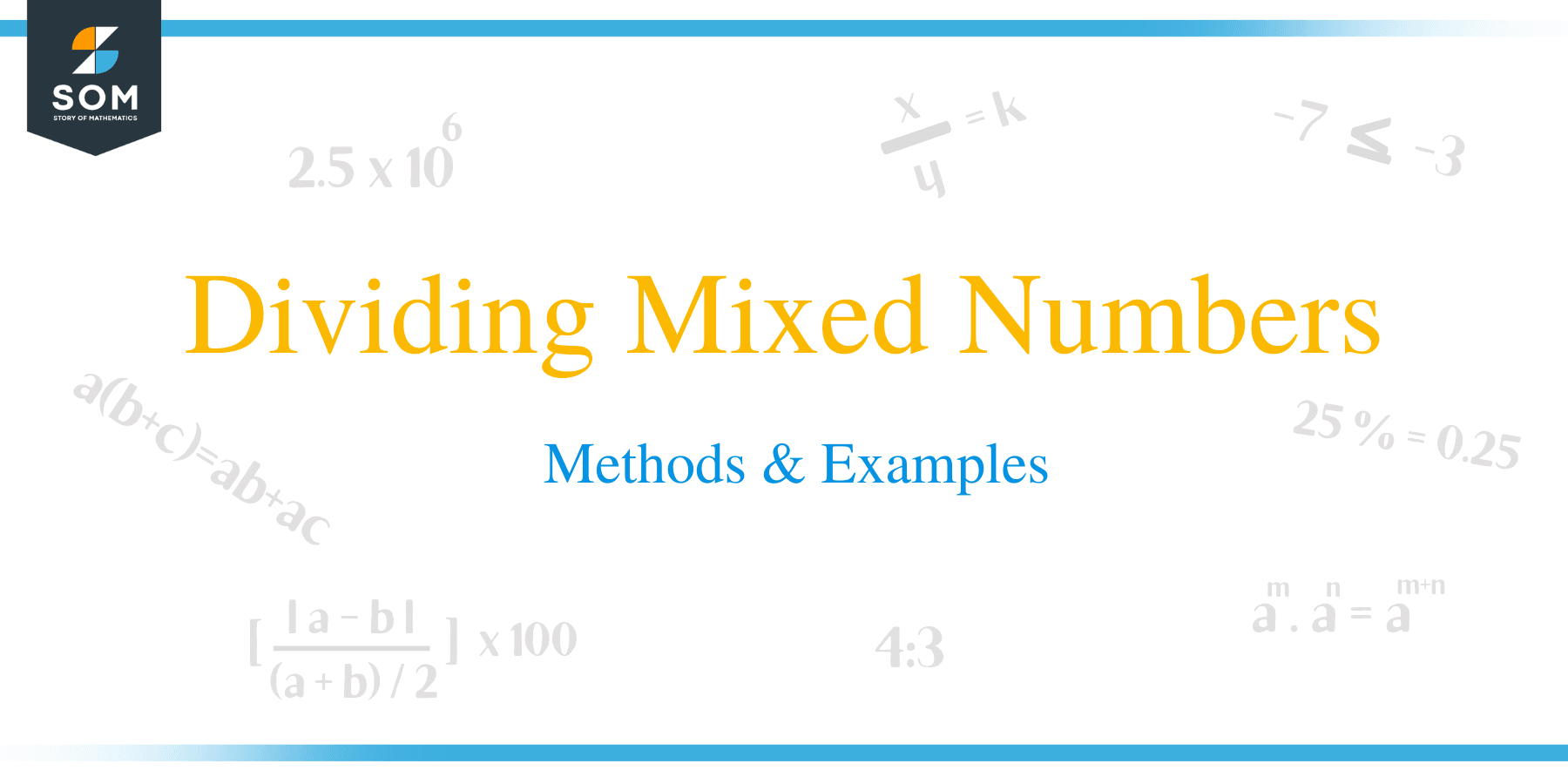# Dividing Mixed Numbers – Methods & Examples

##How to Divide Mixed Numbers?

Mixed numbers consist of an integer followed by a fraction. It is initially an improper fraction, which then broken down into a mixed number form. Division of mixed numbers is very similar to the multiplication of the mixed numbers.

Here are the steps followed when dividing mixed numbers:

• Begin by converting each mixed fraction to an improper.
• Invert or flip upside down the improper fraction that is the divisor
• Multiply the first fraction by the second fraction. Multiplication of numerators and denominators are done separately.
• Convert the resultant fraction into a mixed number if it is improper.
• Simplify the mixed number to its lowest terms possible.

Example 1

Solve the following

1 3/4 ÷ 2 2/5

Solution

• Convert each mixed number into improper fraction.

1 3/4 = 7/4 and 2 2/5 = 12/5

• Now proceed with the division as:

1 3/4 ÷ 2 2/5 = 7/4 ÷ 12/5

• Determine the reciprocal of the second fraction as 5/12

7/4 ÷ 12/5 = 7/4 x 5/12

• Multiply the numerators together and denominators also together.

7/4 x 5/12= (5 x 7)/(12 x 4)

= 35/48Example 2

Workout:

2 ¾ ÷ 1 2/3

Solution

2 ¾ ÷ 1 2/3

= 11/4 ÷ 5/3

= 11/4 × 3/5

= (11 × 3)/(4 × 5)

= 33/20

= 1 13/20

Example 3

Simplify the following,

4/17 ÷ 1 4/17

Solution

4/17 ÷ 1 4/17

= 38/17 ÷ 21/17

= 38/17 × 17/21

= (38 × 17)/(17 × 21)

= 646/357

= 38/21

= 1 17/21

Example 4

Work out: 3 1/3 ÷ 1 5/6

Solution

Step 1:

Convert each mixed number to an improper fraction.

3 1/3 = 10/3 and 1 5/6 = 11/6

Now, 3 1/3 ÷ 1 5/6 = 10/3 ÷ 11/6

Step 2:

Invert the second fraction and change the operator to multiplication.

10/3 ÷ 11/6 = 10/3 x 6/11

Step 3:

Multiply the numerators at the top and denominators at the bottom.

10/3 x 6/11 = (10 x 6)/(11 x 3)

= 60/33

Step 4:

Both the numerator and denominator have a common factor 3, and therefore simplify the fraction to its lowest terms.

60/33 = 20/11

Now convert the answer back to a mixed number.

20/11= 1 9/11

Therefore, 3 1/3 ÷ 1 5/6 = 1 9/11

Example 5

Work out: 4 ÷ 2 1/3

Solution

Step 1:

Convert the mixed numbers into improper fractions.

2 1/3 = 7/3

4 ÷ 2 1/3 = 4/1÷ 7/3

Step 2:

Find the reciprocal of the second fraction and change the operator to multiplication.

4/1÷ 7/3 = 4/1 x 3/7

Step 3:

Multiply the fractions

4 × 3/7 = 12/7

Step 4:

Simplify and convert.

Now convert the fraction back to a mixed number.

12/7 = 1 5/7

Example 6

Two number have a product of 18. If one number is 8 2/5, Calculate the value of the other number.

Solution

The product of the numbers = 18

One of the numbers = 8 2/5 = {(8 × 5) + 2}/5 = 42/5

To find the value of the other number, divide 18 by the fraction.

= 18 ÷ 42/5 = 18 × 5/42

= 90/42

= 15/7

Therefore, the other number is:

= 2 1/7

Example 7

A 25 m long pole is cut into logs of each 1 2/3 meters. Calculate the total number of logs cut from the pole.

Solution

Total number of logs cut can be calculated by dividing 25 m by 1 2/3 = 25 ÷ 1 2/3

= 25 ÷ 5/3

= 25 × 3/5

= 75/5

Therefore, the number of logs cut = 15

### Practice Questions

1. Two numbers, $x$ and $y$, when multiplied together, the result is $\dfrac{1 1}{17}$. If $y= \dfrac{17}{5}$, what is the value of $x$?

2. An athlete runs $3 \dfrac{1}{7}$ km in $1 \dfrac{1}{4}$. How far can he cover if runs at the same speed in an hour?

3. Rex paints $\dfrac{3}{4}$ of a wall in $1 \dfrac{2}{3}$ hours.  How many more hours does he need to complete painting the wall?

4. Mike cut $1 \dfrac{1}{17}$ meters rope into pieces of $\dfrac{2}{17}$ m each. What is the total number of pieces that were cut?

5. A boy completes $\dfrac{2}{3}$ of a work in $25 \dfrac{1}{2}$ hours. What is the total number of hours needed to complete the whole work?

6. A student reads one-third of a book in $2 \dfrac{1}{7}$ hours. How many more hours are needed in order for the student to complete reading the whole book?